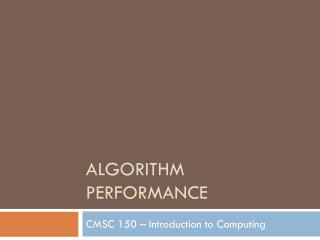DownloadDownload PresentationAlgorithm Performance

# Algorithm Performance

Download Presentation## Algorithm Performance

- - - - - - - - - - - - - - - - - - - - - - - - - - - E N D - - - - - - - - - - - - - - - - - - - - - - - - - - -
##### Presentation Transcript

1. Algorithm Performance CMSC 150 – Introduction to Computing

2. Big-Oh: Algorithm Complexity • We group algorithms into classes of similar complexity (i.e., how many steps required) • Sequential search is a O(n) algorithm (linear) O(1) O(logn) O(n) O(n2) O(2n) Sequential search Modifying a 2D image Traveling Salesman (brute force) constant-time logarithmic linear quadratic exponential

3. Some Comparisons • nis the size of the input • Entry in table gives approximate number of steps required by an algorithm of that complexity

4. Analyzing binary search • Consider worst case for an array of size 32: 32 elements 16 elements 8 elements 4 elements 2 elements 1 element

5. Analyzing binary search • Consider worst case for an array of size 32: 32 elements 16 elements 8 elements • Ignore the last (1-elementarray) comparison • Start with 32 elements • 5 comparisons total until found • Note 25 = 32  log2 32 = 5 • Log2 of input size gives ~ number of comparisons 4 elements 2 elements 1 element

6. Big-Oh: Algorithm Complexity • Binary search is a O(logn) algorithm (logarithmic) O(1) O(logn) O(n) O(n2) O(2n) Binary search Sequential search Modifying a 2D image Traveling Salesman (brute force) constant-time logarithmic linear quadratic exponential

7. Some Comparisons • nis the size of the input binary search sequential search

8. Sequential vs. Binary Search • Sequential • O(n): linear • Easy to implement • Inefficient for large input size • Binary: O(logn) • O(logn): logarithmic • Reasonably efficient for large input size • Requires the list to be sorted